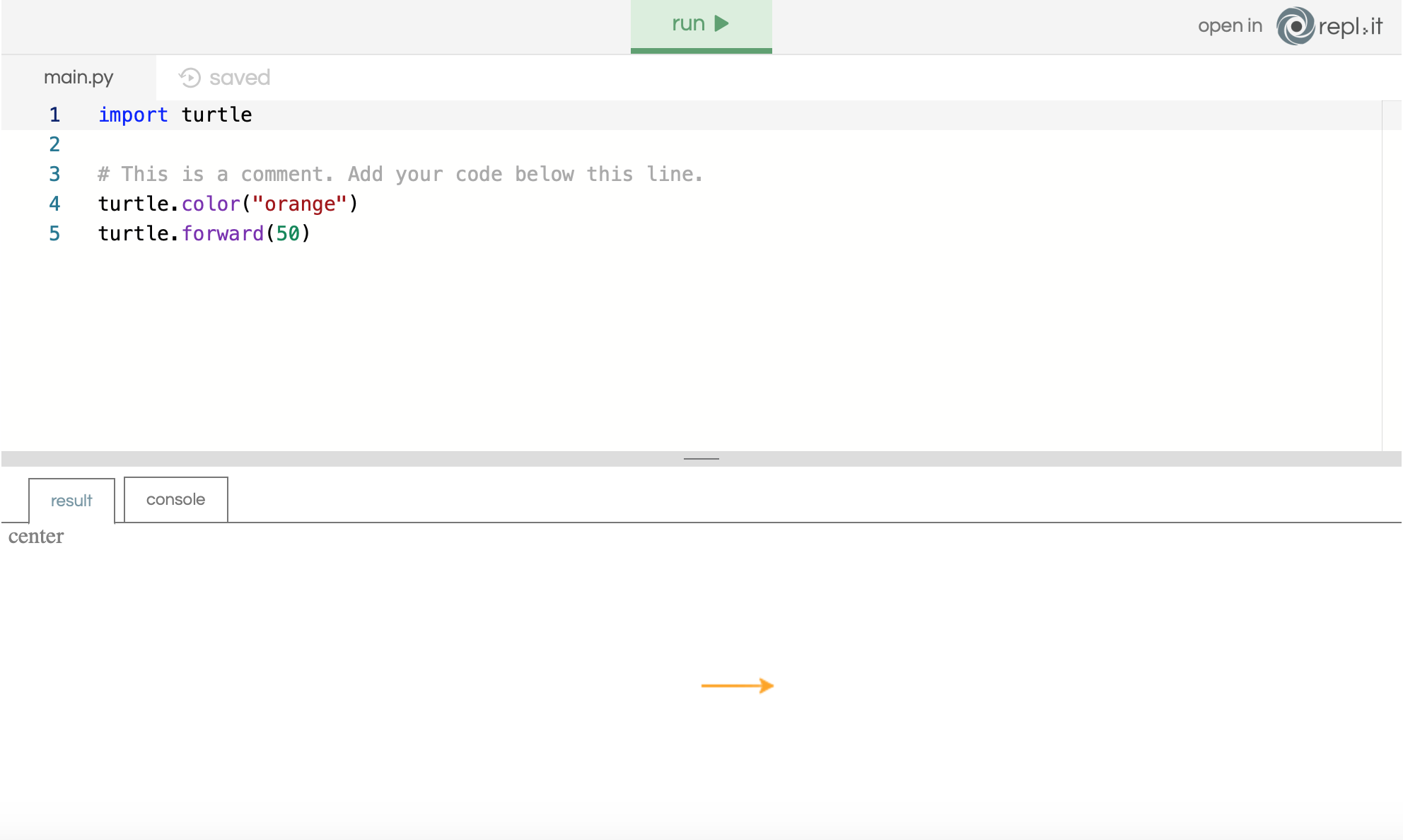# The Basics - Drawing a colored line

Before Alex can learn how to create a beehive, he must first learn how to draw a colored line. We will add code to the below repl.it window, and we will do this in two parts.

First, we need tell the turtle what color we want the line to be. To do this, let’s type in:

``````turtle.color("orange")
``````

Let’s try to understand this line of code in more detail.

Now that we have picked the color that we want, we can now draw a line! To draw a line there are two methods that we can use:

``````turtle.forward(50)
turtle.backward(50)
``````

`turtle.forward(50)` will make the turtle go forward 50 pixels and draw a line in the direction it’s facing, while `turtle.backward(50)` will do the same, but in the opposite direction.

Let’s make the turtle go forward, so type in `turtle.forward(50)`. Now your code should look something like this:

``````import turtle

turtle.color("orange")
turtle.forward(50)
``````

Now that we have completed our code, press run. You should see the following in the result screen:``````turtle.shape("turtle")